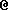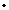PEOPLE Associates
Institut des Hautes Études Scientifiques, France
Area of Research:  Probability and Mathematical Physics
goswamiihesfr

Probability theory and its applications to problems in statistical physics and statistics. Broadly speaking, I am interested in studying geometrical and topological properties of large random sets. Some of my current projects involve understanding percolation phenomena in correlated media like random cluster models, random interlacement and level-sets of Gaussian free field among others. I am also very interested in mathematical statistics, specifically the interface of probability theory, statistics and optimization